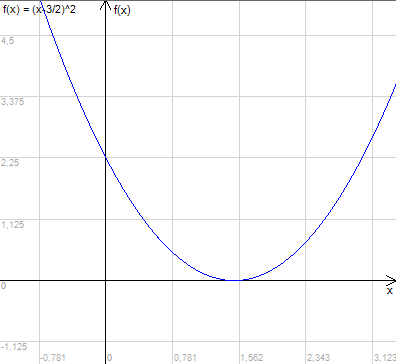### Features And Algorithms

This library uses numerical calculator library so can handle same functions at input.

Algorithm used in library is a hybrid of bisection method and newton method. Thanks to that algorithm won't fail even searching roots for x^2 function and similar.

### Example and usage

Take function f(x)=(x-3/2)^2

Graph of this function shows that the root is somewhere between points 1 and 2:You can use this library to find exact point:

`string function = "(x-3/2)^2";double xFrom = 1d;double xTo = 2d;Hybrid hybrid = new Hybrid(function, xFrom, xTo);double result = hybrid.ComputeHybrid();`

### Example application